# Calculator system of equations with imaginary numbers

Input contains an equal sign thus computation continues to equation (or system of equations or quadratic equation or cubic equation).

### Solution:

System of equations are non-linear.
This calculator does basic arithmetic on complex numbers and evaluates expressions in the set of complex numbers. As imaginary unit use i or j (in electrical engineering), which satisfies basic equation i2 = −1 or j2 = −1. The calculator also provides conversion of a complex number into angle notation (phasor notation), exponential, or polar coordinates (magnitude and angle). Enter expression with complex numbers like 5*(1+i)(-2-5i)^2

Complex numbers in the angle notation or phasor (polar coordinates r, θ) may you write as rLθ where r is magnitude/amplitude/radius, and θ is angle (phase) in degrees, for example, 5L65 which is same as 5*cis(65°).
Example of multiplication of two imaginary numbers in the angle/polar/phasor notation: 10L45 * 3L90.

Why the next complex numbers calculator when we have WolframAlpha? Because Wolfram tool is slow and some features such as step by step are charged premium service.
For use in education (for example, calculations of alternating currents at high school), you need a quick and precise complex number calculator.

## Basic operations with complex numbers

We hope that work with the complex number is quite easy because you can work with imaginary unit i as a variable. And use definition i2 = -1 to simplify complex expressions. Many operations are the same as operations with two-dimensional vectors.

Very simple, add up the real parts (without i) and add up the imaginary parts (with i):
This is equal to use rule: (a+bi)+(c+di) = (a+c) + (b+d)i

(1+i) + (6-5i) = 7-4i
12 + 6-5i = 18-5i
(10-5i) + (-5+5i) = 5

### Subtraction

Again very simple, subtract the real parts and subtract the imaginary parts (with i):
This is equal to use rule: (a+bi)+(c+di) = (a-c) + (b-d)i

(1+i) - (3-5i) = -2+6i
-1/2 - (6-5i) = -6.5+5i
(10-5i) - (-5+5i) = 15-10i

### Multiplication

To multiply two complex number use distributive law, avoid binomials and apply i2 = -1.
This is equal to use rule: (a+bi)(c+di) = (ac-bd) + (ad+bc)i

(1+i) (3+5i) = 1*3+1*5i+i*3+i*5i = 3+5i+3i-5 = -2+8i
-1/2 * (6-5i) = -3+2.5i
(10-5i) * (-5+5i) = -25+75i

### Division

Division of two complex number is based on avoid imaginary unit i from denominator. This can be done only via i2 = -1. If denominator is c+di, to make it without i (or make it real), just multiply with conjugate c-di:

(c+di)(c-di) = c2+d2

(10-5i) / (1+i) = 2.5-7.5i
-3 / (2-i) = -1.2-0.6i
6i / (4+3i) = 0.72+0.96i

### Absolute value or modulus

Absolute value or modulus is distance of image of complex number from origin in plane. That use Pythagorean theorem, just as case of 2D vector. Very simple, see examples: |3+4i| = 5
|1-i| = 1.4142136
|6i| = 6
abs(2+5i) = 5.3851648

### Square root

Square root of complex number (a+bi) is z, if z2 = (a+bi). Here ends simplicity. Because of the fundamental theorem of algebra, you will always have two different square roots for a given number. If you want to find out the possible values, the easiest way is probably to go with De Moivre's formula. Here our calculator is on edge, because square root is not a well defined function on complex number. We calculate all complex roots from any number - even in expressions:

sqrt(9i) = 2.1213203+2.1213203i
sqrt(10-6i) = 3.2910412-0.9115656i
pow(-32,1/5)/5 = -0.4
pow(1+2i,1/3)*sqrt(4) = 2.439233+0.9434225i
pow(-5i,1/8)*pow(8,1/3) = 2.3986959-0.4771303i

### Square, power, complex exponentiation

Yes, our calculator can power any complex number to any integer (positive, negative), real or even complex number. In another words, we calculate 'complex number to a complex power' or 'complex number raised to a power'...
Famous example:
$i^i = e^{-\pi/2}$
i^2 = -1
i^61 = i
(6-2i)^6 = -22528-59904i
(6-i)^4.5 = 2486.1377428-2284.5557378i
(6-5i)^(-3+32i) = 2929449.03994-9022199.58262i
i^i = 0.2078795764
pow(1+i,3) = -2+2i

### Functions

sqrt
Square Root of a value or expression.
sin
sine of a value or expression. Autodetect radians/degrees.
cos
cosine of a value or expression. Autodetect radians/degrees.
tan/tg
tangent of a value or expression. Autodetect radians/degrees.
exp
e (the Euler Constant) raised to the power of a value or expression
pow
Power one complex number to another integer/real/comple number
ln
The natural logarithm of a value or expression
log
The base-10 logarithm of a value or expression
abs or |1+i|
Absolute value of a value or expression
phase
Phase (angle) of a complex number
cis
is less known notation: cis(x) = cos(x)+ i sin(x); example: cis (pi/2) + 3 = 3+i
conj
conjugate of complex number - example: conj(4i+5) = 5-4i

## Complex numbers in word problems:

• Is complexAre these numbers 2i, 4i, 2i + 1, 8i, 2i + 3, 4 + 7i, 8i, 8i + 4, 5i, 6i, 3i complex?
• BearingA plane flew 50 km on a bearing 63°20' and the flew on a bearing 153°20' for 140km. Find the distance between the starting point and the ending point.
• Log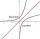Calculate value of expression log |3 +7i +5i2| .
• Imaginary numbersFind two imaginary numbers whose sum is a real number. How are the two imaginary numbers related? What is its sum?
• ABS CNCalculate the absolute value of complex number -15-29i.
• ReciprocalCalculate reciprocal of z=0.8-1.8i:A domed stadium is in the shape of spherical segment with a base radius of 150 m. The dome must contain a volume of 3500000 m³. Determine the height of the dome at its centre to the nearest tenth of a meter.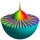Let z1=x1+y1i and z2=x2+y2i Find: a = Im (z1z2) b = Re (z1/z2)Which coordinates show the location of -2+3i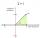Is -10i a positive number?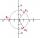Find the cube roots of 125(cos 288° + i sin 288°).Given that ? "this is z star" Find the value of the complex number z.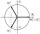There are two distinct complex numbers z such that z3 is equal to 1 and z is not equal 1. Calculate the sum of these two numbers.